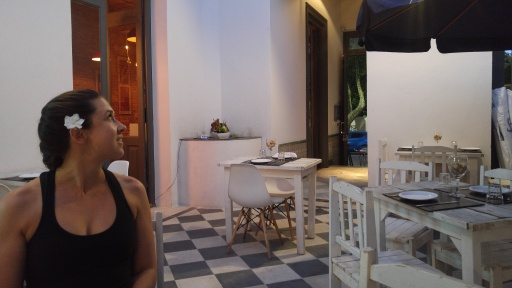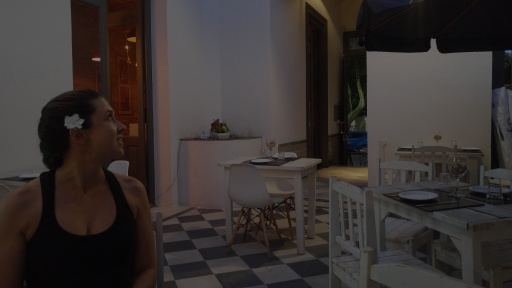2018-10-29

## Putting Some Pieces Together: OpenCV

OpenCV is the foremost image processing library on the planet.

Disagree?

Most, if not all, machine learning systems that deal with images use it. Its bevy of interesting algorithms go far beyond raw image manipulation, and it is really good at raw image manipulation. So, regardless of whether the unknowable claim of foremost above could be true, it is our considered position that OpenCV makes all of the legacy Java image processing fairly pointless. Especially if what you are doing is actually processing images (as opposed to setting icons on awt widgets).

The problem with OpenCV, from a Java perspective, really lies in needing to learn a set of custom interfaces to do non-custom things.

Oh, and dealing with native libraries...

Now we can see an interesting application of those two: tech.opencv.

#### Image Joy!

``````user> (require '[tech.opencv :as opencv])
WARNING: cast already refers to: #'clojure.core/cast in namespace: tech.datatype.base, being replaced by: #'tech.datatype.base/cast
WARNING: cast already refers to: #'clojure.core/cast in namespace: tech.datatype, being replaced by: #'tech.datatype/cast
nil
#<org.bytedeco.javacpp.opencv_core\$Mat@28a27eb4 org.bytedeco.javacpp.opencv_core\$Mat[width=512,height=288,depth=8,channels=3]>
user> (def test-img *1)
#'user/test-img
``````We got back an `opencv::mat` in the form of a JavaCPP pointer. Now what can we do with it?

``````user> (require '[clojure.core.matrix :as m])
nil
user> (m/ecount test-img)
442368
user> (require '[tech.datatype :as dtype])
nil
user> (dtype/get-datatype test-img)
:uint8
user> (m/shape test-img)
[288 512 3]
user> (def short-data (short-array (m/ecount test-img)))
#'user/short-data
user> (dtype/copy! test-img short-data)
#<[S@65be63b7>
user> (take 20 short-data)
(172 170 170 172 170 170 171 169 169 171 169 169 173 171 171 174 172 172 174 172)
user> ;; Note correct representation of data.
``````

It should be noted that `shape` and `ecount` are really all `core.matrix` provides at this point. Over the next few posts, we will implement a bit more. That being said, you still have access to all of OpenCV.

`m/shape` gets you further than you might think:

``````user> (m/shape test-img)
[288 512 3]
user> (m/shape [test-img test-img test-img])
[3 288 512 3]
user> (m/shape (repeat 10 [test-img test-img test-img]))
[10 3 288 512 3]
``````

Copy of course works both ways:

``````user> (require '[clojure.core.matrix.macros :refer [c-for]])
nil
user> (c-for [idx (int 0) (< idx (m/ecount test-img)) (inc idx)]
(aset ^shorts short-data idx (short (quot (aget ^shorts short-data idx) 2))))
nil
user> (def dest-img (opencv/new-mat 288 512 3))
#'user/dest-img
user> (opencv/save dest-img "darken.png")
#<org.bytedeco.javacpp.opencv_core\$Mat@4674cb52 org.bytedeco.javacpp.opencv_core\$Mat[width=512,height=288,depth=8,channels=3]>
``````

Here, `quot 2` brings the data closer to zero, darkening the image.The resource system is also in play, so matrices allocated within a resource context will be released when the resource context unwinds:

``````user> (require '[tech.resource :as resource])
nil
user> (resource/with-resource-context
test-img))
``````

Note that pointer address is zero.

You also have access to OpenCV's methods, but so-far they are not wrapped nicely.

``````user> (import '[org.bytedeco.javacpp opencv_imgproc opencv_core opencv_core\$Mat])
#<Class@16b65cc1 org.bytedeco.javacpp.opencv_core\$Mat>
#'user/test-img
user> (def result-img (opencv/clone test-img))
#'user/result-img
user> (opencv_imgproc/blur test-img result-img (opencv/size 3 3))
nil
user> (opencv/save result-img "blurry.png")
#<org.bytedeco.javacpp.opencv_core\$Mat@6330f6da org.bytedeco.javacpp.opencv_core\$Mat[width=512,height=288,depth=8,channels=3]>
user>
``````They are, however, quite fast:

``````user> (time (dotimes [iter 100]
(opencv_imgproc/blur test-img result-img (opencv/size 3 3))))
"Elapsed time: 73.80491 msecs"
nil
``````

#### Some Implementation Details

A simple conversion between an OpenCV matrix and a typed pointer (of the type of data in the matrix) drastically simplifies this kind of interop. With our system, all the intense code required to correctly marshal information into and out of OpenCV can be activated by 3 protocol methods.

``````tech.datatype.primitive/PBuffer
tech.datatype.base/PDatatype
clojure.core.matrix.protocols/PElementCount
``````

``````tech.datatype.base/PContainerType
``````

The OpenCV matrix class partially satisfies these with its Pointer class, and it has a `.ptr` member variable that points to the actual image data. Finishing the implementation on top of OpenCV's additional datatypes requires a few more functions:

``````(extend-type opencv_core\$Mat
resource/PResource
(release-resource [item] (.release item) (.deallocate item))
mp/PDimensionInfo
(dimensionality [m] (count (mp/get-shape m)))
(get-shape [m] [(.rows m) (.cols m) (.channels m)])
(is-scalar? [m] false)
(is-vector? [m] true)
(dimension-count [m dimension-number]
(let [shape (mp/get-shape m)]
(if (<= (count shape) (long dimension-number))
(get shape dimension-number)
(throw (ex-info "Array does not have specific dimension"
{:dimension-number dimension-number
:shape shape})))))
mp/PElementCount
(element-count [m] (apply * (mp/get-shape m)))

dtype/PDatatype
(get-datatype [m] (-> (.type m)
opencv-type->channels-datatype
:datatype))

jcpp-dtype/PToPtr
(->ptr-backing-store [item] (jcpp-dtype/set-pointer-limit-and-capacity
(.ptr item)
(mp/element-count item)))
;;We gain a lot from inheritance of the base Pointer type (opencv_core\$Mat inherits
;;from javacpp/Pointer.  This allows all the machinery required to interact
;;with the datatype copy system to be implemented once in Pointer.
)
``````

The last bit, copy raw data, is there to allow this to be used in sequences. For instance, converting a batch of 10 images into a single floating point of data to be used in a neural networking inference or training run:

``````(def test-ary (float-array (* 3 (m/ecount test-img))))
#'user/test-ary
user> (dtype/copy-raw->item! (repeat 3 test-img) test-ary 0)
[#<[F@5ef59d3c> 1327104]
user> (take 10 test-ary)
(172.0 170.0 170.0 172.0 170.0 170.0 171.0 169.0 169.0 171.0)
``````

The full code is around 250 lines:

We are leveraging our architecture that provides far easier integration of bulk primitive datatypes with native applications. So everything in this post can be short, simple, and exhibit predictable behavior.

#### Computing With Images

We have another library, tech.compute. This library provides substantial infrastructure for doing the types of math computations that come up all the time in our work. There is a lot to it, but suffice it to say that it was carefully engineered to work on native datatypes as well as JVM datatypes to make interoperability transparent. With these tools we can do (among other things) linear algebra operations directly with opencv images without copying the underlying buffer data. (!)

Here is the above example (darken by dividing by 2) written with the compute library:

``````;; Load CPU layer of compute library.
user> (require '[tech.compute.cpu.tensor-math :as cpu-tm])
nil
user> (require '[tech.compute.tensor.operations :as op])
nil
(op// 2)
(opencv/save "tensor_darken.jpg"))
#<org.bytedeco.javacpp.opencv_core\$Mat@1083378d org.bytedeco.javacpp.opencv_core\$Mat[width=512,height=288,depth=8,channels=3]>
``````

Nice.RGB to BGR conversion serves as a slightly more extensive example. Doing rgb->bgr translations or normalizing image data to be in a different range are just some of the extremely common things we ran into when working with images. We wish we would have had this library from the beginning!

At TechAscent, we love this shit!